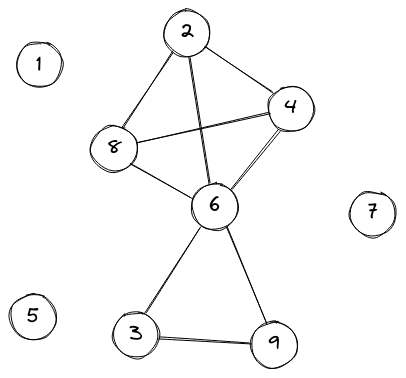# Hidden GraphPeregrine_FalconNational High School Prog...
Limits 2s, 512 MB

There is an unweighted, undirected hidden graph. You are given an integer range from $L$ to $R$. There are total of$R-L+1$ nodes numbered from $L$ to $R$. Two nodes $U,V$ have an edge between them if the $GCD(U, V)$ is greater than $1$.

You have to find the number of the connected components $X$, of this graph and find the size of each component.

An explanation of the unweighted and undirected graph can be found here. An explanation of the connected component can be found here.

## Input

The first line of the input consists of the number of test case $T$ $(1 \leq T \leq 10)$. Each test case consists of two integers $L$ and $R$.

For 40 Points: $(1 \leq L \leq R \leq 10^3)$.
For 100 Points: $(1 \leq L \leq R \leq 10^6)$.

## Output

The first line of each test case consists of integer $X$ denoting the number of connected components of the graph. Then each of the next X lines consists of the size of each component in sorted order.

## Sample

InputOutput
2
1 9
2 7

4
1
1
1
6
3
1
1
4



Hidden graph for the first test case:The greatest common divisor for the following pairs is greater than 1: $(2,4)$, $(2,6)$, $(2,8)$, $(4,6)$, $(4,8)$, $(6,8)$, $(3,6)$, $(3,9)$, $(6,9)$.

Each pair of nodes above share a common edge between them. So, there are 3 components consists of 1 node and another component with 6 nodes.uDebug

### Submit